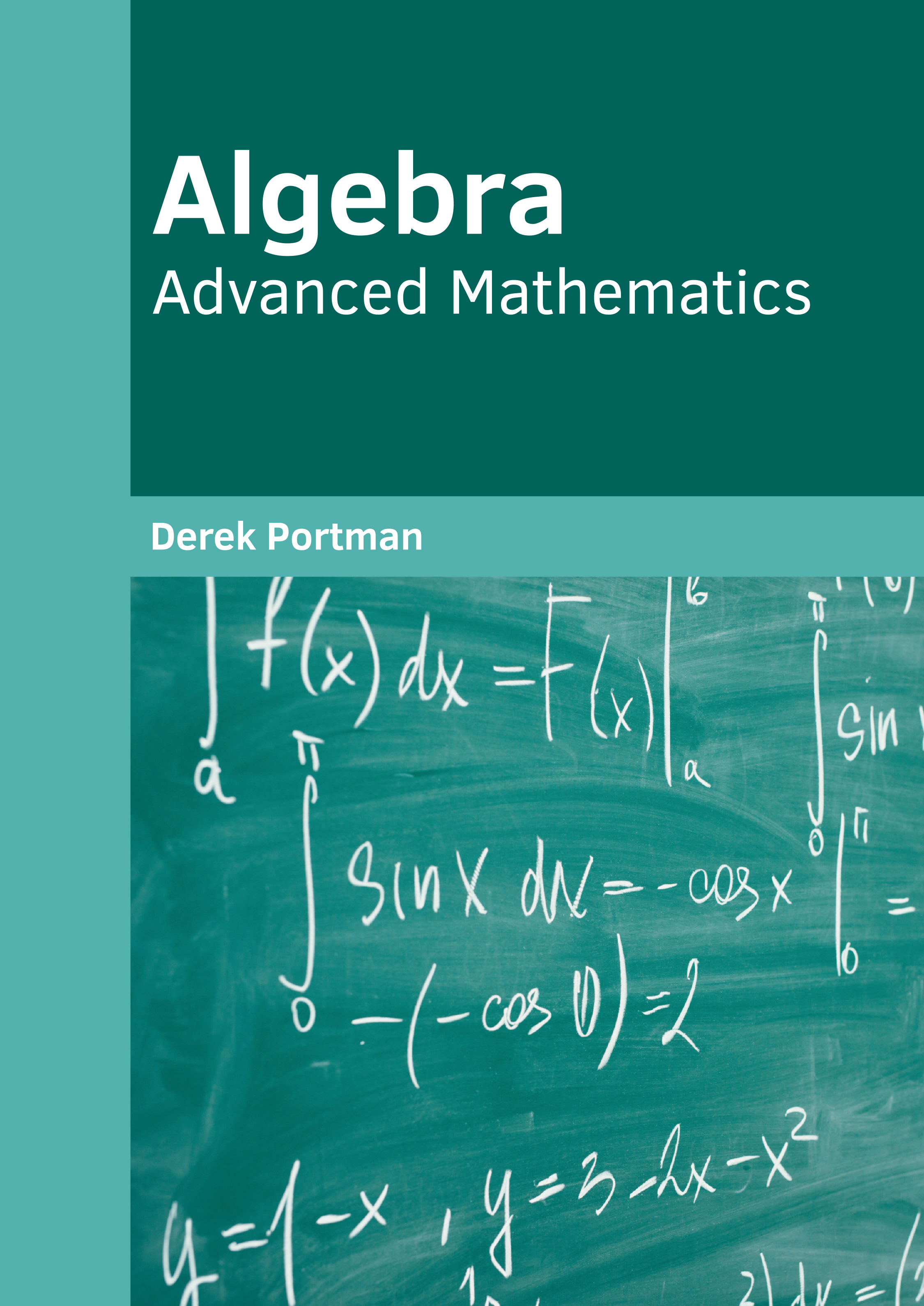• library@msu.ac.th
• Academic Resource Center Mahasarakham University
• library@msu.ac.th
• Academic Resource Center Mahasarakham Universityหมวดหมู่Algebra is one of the major branches of mathematics which studies mathematical symbols and the rules used for handling these symbols. It deals with numerous concepts such as elementary equations and abstractions such as groups, rings and fields. There are two subdomains within algebra, namely, elementary algebra and abstract algebra. Elementary algebra is the basic part of algebra and it is used in different branches of mathematics, science, engineering, medicine and economics. Advanced mathematics is a major area in abstract algebra. This book provides comprehensive insights into the field of algebra. The various advancements in this discipline are glanced at and their applications as well as ramifications are looked at in detail. The extensive content of this book provides the readers with a thorough understanding of the subject

ใส่ตะกร้า
• ISBN9781682858257
• ประเภท E-Book
• ผู้แต่ง Derek Portman,
• สำนักพิมพ์ Willford
• ครั้งที่พิมพ์ 1
• ปีที่พิมพ์2020
• ภาษาภาษาอังกฤษ
• หมวดหมู่วิทยาศาสตร์
###### : ข้อมูลหนังสือ

Algebra is one of the major branches of mathematics which studies mathematical symbols and the rules used for handling these symbols. It deals with numerous concepts such as elementary equations and abstractions such as groups, rings and fields. There are two subdomains within algebra, namely, elementary algebra and abstract algebra. Elementary algebra is the basic part of algebra and it is used in different branches of mathematics, science, engineering, medicine and economics. Advanced mathematics is a major area in abstract algebra. This book provides comprehensive insights into the field of algebra. The various advancements in this discipline are glanced at and their applications as well as ramifications are looked at in detail. The extensive content of this book provides the readers with a thorough understanding of the subject